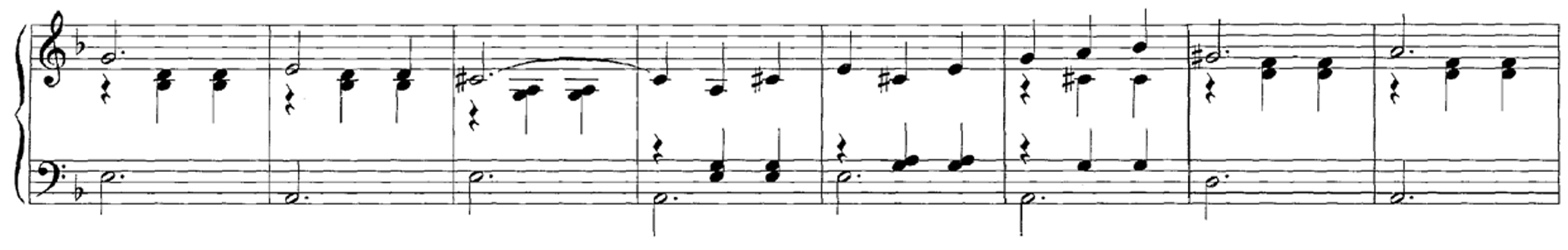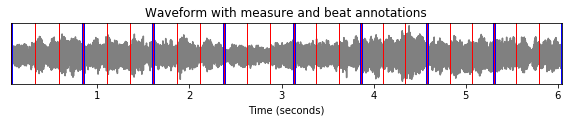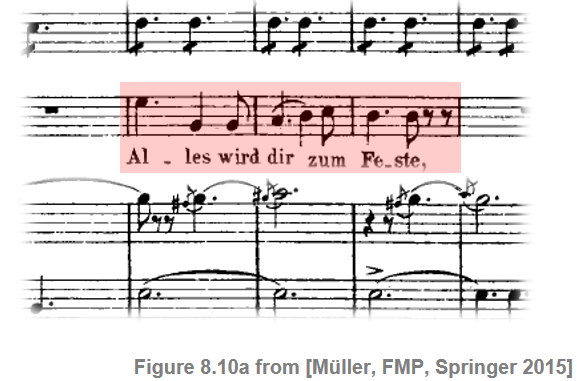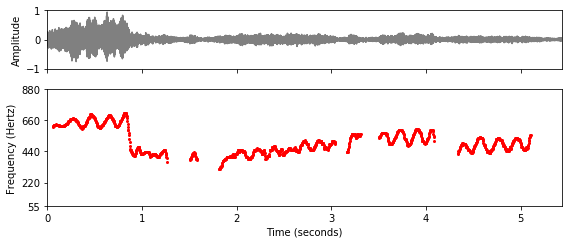# Sonification

In this notebook, we introduce some tools that can be used for the sonification of feature representations and annotations.

## Introduction¶

In the FMP notebooks, we use a multitude of figures with concrete examples to communicate the main ideas of music processing approaches. In particular, the visualization of feature representations such as spectrograms, chromagrams, or tempograms not only deepens the understanding of signal processing concepts, but also provides valuable insights into musical and acoustic properties of the underlying audio signals. Furthermore, the combined visualization of extracted features and reference annotations allows for an in-depth inspection of algorithmic approaches on a piece-wise level. Such qualitative evaluations are, besides quantitative evaluations based on suitable metrics, essential for understanding the benefits and limitations of algorithmic approaches as well as the suitability and validity of the underlying model assumptions.

As an alternative or complement to data visualization, one may also use data sonification as a means for providing acoustic feedback on the nature of extracted or annotated information. This particularly holds for music, where humans are trained to perceive even slight deviations in frequency and timing of sound events. For example, one can immediately recognize small rhythmic deviations when listing to a pulse track sonified in form a sequence of click sounds. In this notebook, we introduce three sonification methods that are helpful in analyzing annotations as well as audio features extracted from a music recording.

Obviously, there are many more ways for sonifying acoustic properties of music signals. In particular, a superposition of the original music signal and a suitable sonification of specific features often leads to fascinating and surprising insights.

## Sonification of Time Positions¶

In the first scenario, we assume that we are given a music recording as well as a list of time positions that indicate the presence of certain musical events. For example, the musical events may refer to onset positions of certain notes, to beat positions, or structural boundaries between musical parts. Then, the goal of sonification is to generate a succinct acoustic stimulus at each of the time positions, thus giving the listener a precise temporal feedback. Ideally, the stimuli should be perceivable also when being superimposed with the original music recording. Often the time positions are further classified according to different categories (e.g., downbeat and upbeat positions). Therefore, it is useful to have a "coloration" method for generating distinguishable stimuli that can easily be associated with the different categories.

The LibROSA python package provides the function librosa.clicks for generating a family of distinguishable stimuli in form of click sounds that can be placed at the time positions specified. The function allows for adjusting the frequency (with a default of $1000~\mathrm{Hz}$) as well as the duration (with a default of $100~\mathrm{ms}$) of the click signal.

As an example, we consider a short excerpt of an orchestra recording of the Waltz No. 2 from the Suite for Variety Orchestra No. 1 by Dimitri Shostakovich. In the annotation file, we have marked the beat positions (given in seconds). Being in the $3/4$ meter, every third beat corresponds to a downbeat or measure position. In the following code cell, we generate a sonification, where we use a long click sound of low frequency to indicate measure positions and a short click sound of high frequency to indicate beat positions. While, in the visualization, the annotated time positions are superimposed with the waveform plot, the generated click sounds are superimposed with the original music recording.In :
import os
import sys
import numpy as np
import librosa
import pandas as pd
from matplotlib import pyplot as plt
import IPython.display as ipd

sys.path.append('..')
import libfmp.b
import libfmp.c8

%matplotlib inline

fn_wav = os.path.join('..', 'data', 'B', 'FMP_B_Sonify_Beat_Shostakovich_Waltz.wav')
fn_ann = os.path.join('..', 'data', 'B', 'FMP_B_Sonify_Beat_Shostakovich_Waltz.csv')
Fs = 22050
beat_sec = df.values
meas_sec = beat_sec[::3]
ann_beat = [(pos, 'beat') for pos in beat_sec]
ann_meas = [(pos, 'measure') for pos in meas_sec]

# Plot waveform and annotations
label_keys = {'measure': {'linewidth': 3, 'color': 'b'},
'beat': {'linewidth': 1, 'color': 'r'}}
title = 'Waveform with measure and beat annotations'
fig, ax, line = libfmp.b.plot_signal(x, Fs, title=title, figsize=(8, 1.8))
libfmp.b.plot_annotation_line(ann_meas+ann_beat, ax=ax, label_keys=label_keys)
plt.tight_layout()

plt.show()

# Sonify beat and measure annotations
x_beat = librosa.clicks(beat_sec, sr=Fs, click_freq=2000,
length=len(x), click_duration=0.1)
x_meas = librosa.clicks(meas_sec, sr=Fs, click_freq=400,
length=len(x), click_duration=0.3)
ipd.display(ipd.Audio(x + x_beat + x_meas, rate=Fs))## Sonification of Frequency Trajectories¶

When asked to describe a specific song, we are often able to sing or hum the main melody, which may be loosely defined as a linear succession of musical tones expressing a particular musical idea. Given a music recording (rather than a musical score), the melody corresponds to a sequence of fundamental frequency values (also called F0-values) of the tones' pitches. In real performances, such sequences often form complex time–frequency patterns referred to as a frequency trajectories. These trajectories may comprise continuous frequency glides from one to the next note (glissando) or frequency modulations (vibrato). As an example, we consider a short excerpt of an aria from the opera "Der Freischütz" by Carl Maria von Weber. In the score representation, the main melody is notated in a separate staff line underlaid with lyrics.In the performance by a soprano singer, the melody corresponds to an F0-trajectory, which we visualize in the following code cell along with the recording's waveform. Furthermore, we use the function libfmp.c8.sonify_trajectory_with_sinusoid to sonify the F0-trajectory using a sinusoidal synthesizer. The sonification is provided in three different formats:

• As mono signal.
• As mono signal superimposed with the original recording.
• As stereo signal with the F0-sonification in the right and the original recording in the left channel.

The sonification nicely shows that, as opposed to the notated symbolic representation, the singer smoothly connects some of the notes. Also, one can notice rather pronounced frequency modulations due to vibrato. The superposition with the original recording yields a convenient way to perceptually evaluate the temporal and spectral accuracy of the extracted F0-trajectory.

In :
# Load audio and read F0-trajectory annotations
fn_wav = os.path.join('..', 'data', 'B', 'FMP_B_Sonify_F0_Weber_Freischuetz.wav')
fn_traj = os.path.join('..', 'data', 'B', 'FMP_B_Sonify_F0_Weber_Freischuetz.csv')
Fs = 22050
traj = traj_df.values

# Plot waveform and F0-trajectory annotations
fig, ax = plt.subplots(2, 1, gridspec_kw={'height_ratios': [1, 2]}, sharex=True, figsize=(8, 3.5))
libfmp.b.plot_signal(x, Fs, ax=ax, xlabel='')
ax.set_ylim((-1,1))
ax.set_ylabel('Amplitude')
traj_plot = traj[traj[:, 1] > 0]
ax.plot(traj_plot[:, 0], traj_plot[:, 1], color='r', markersize=4, marker='.', linestyle='')
ax.set_ylim((55, 880))
ax.set_yticks([55, 220, 440, 660, 880])
ax.set_xlim((0, len(x) / Fs))
ax.set_ylabel('Frequency (Hertz)')
ax.set_xlabel('Time (seconds)')
plt.tight_layout()
plt.show()

# Sonify F0 trajectory
x_traj_mono = libfmp.c8.sonify_trajectory_with_sinusoid(traj, len(x), Fs, smooth_len=11, amplitude=0.6)
# left: x, right: sonification
x_traj_stereo = np.vstack((x.reshape(1, -1), x_traj_mono.reshape(1, -1)))
print('F0 sonification (mono)')
ipd.display(ipd.Audio(x_traj_mono, rate=Fs))
print('F0 sonification superimposed with original recording (mono)')
ipd.display(ipd.Audio( (x_traj_mono+x) / 2, rate=Fs))
print('F0 sonification (right channel), original recording (left channel)')
ipd.display(ipd.Audio(x_traj_stereo, rate=Fs))F0 sonification (mono)

F0 sonification superimposed with original recording (mono)